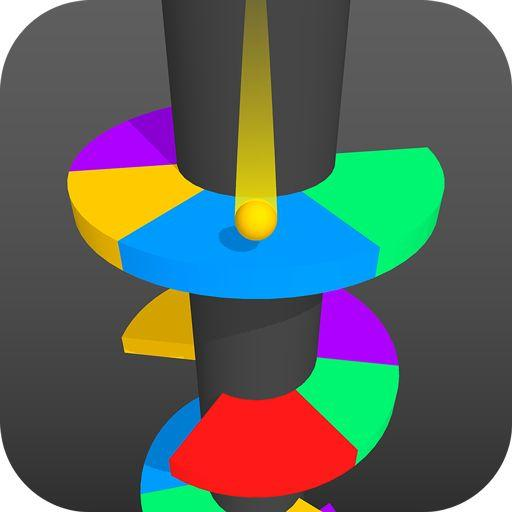........

## 2.使用Mesh实现自定义3d物体

``````using System.Collections;
using System.Collections.Generic;
using UnityEngine;

[RequireComponent(typeof(MeshFilter), typeof(MeshRenderer))]
public class TestDisk : MonoBehaviour
{
MeshFilter MeshFilter;
MeshRenderer MeshRenderer;

public float height = 0.4f;
public int details = 20;

static float EPS = 0.01f;

void Start()
{
MeshFilter = transform.GetComponent<MeshFilter>();
MeshRenderer = transform.GetComponent<MeshRenderer>();

}
//生成一个弧度随机的圆片
{
var arcs = new List<float>();
// 按比例随机，0代表空的，1代表整圆
float r = Random.Range(0.1f, 0.9f);
r *= 2 * Mathf.PI;

GeneratePie(arcs);

}

// 参数：每个弧用两两个弧度（float）表示，每个饼可以有多个三角块，就和切披萨一样
public void GeneratePie(List<float> arcs)
{
List<Vector3> verts = new List<Vector3>();
List<Vector2> uvs = new List<Vector2>();
List<int> tris = new List<int>();

List<Vector3> _verts = new List<Vector3>();
List<Vector2> _uvs = new List<Vector2>();
List<int> _tris = new List<int>();

for (int i = 0; i < arcs.Count; i += 2)
{
_verts.Clear();
_uvs.Clear();
_tris.Clear();

//先把中心点添加进顶点List中

AddArcMeshInfo(arcs[i], arcs[i + 1], _verts, _uvs, _tris);

//把顶点序号填进三角形list里
foreach (int n in _tris)
{
}
}
Mesh mesh = new Mesh();
// 填写mesh
mesh.vertices = verts.ToArray();
mesh.triangles = tris.ToArray();
mesh.uv = uvs.ToArray();

//根据顶点和三角形数据自动生成体积框,法线和切线
mesh.RecalculateBounds();
mesh.RecalculateNormals();
mesh.RecalculateTangents();

MeshFilter.mesh = mesh;

}

void AddArcMeshInfo(float begin, float end, List<Vector3> verts, List<Vector2> uvs, List<int> tris)
{
// begin和end是开始弧度、结束弧度
// verts里面已经有了顶面中心点、底面中心点，下标分别是0和1

float eachRad = 2 * Mathf.PI / details;

// 用三角函数计算出圆的周长上的顶点
float a;
for (a = begin; a <= end; a += eachRad)
{
}
if (a < end + EPS)
{
}

// 顶面顶点序号
int n = verts.Count;
for (int i = 2; i < n - 2; i += 2)
{
}

// 侧面顶点序号
for (int i = 2; i < n - 2; i += 2)
{
}

// 封住两个直线边
}
}
``````

``````    IEnumerator SequenceTest()
{
for (int i = 0; i < MeshFilter.mesh.triangles.Length; i += 3)
{
Debug.DrawLine(MeshFilter.mesh.vertices[MeshFilter.mesh.triangles[i]], MeshFilter.mesh.vertices[MeshFilter.mesh.triangles[i + 1]], Color.red, 100f);

yield return new WaitForSeconds(0.2f);
Debug.DrawLine(MeshFilter.mesh.vertices[MeshFilter.mesh.triangles[i + 1]], MeshFilter.mesh.vertices[MeshFilter.mesh.triangles[i + 2]], Color.yellow, 100f);

yield return new WaitForSeconds(0.2f);
Debug.DrawLine(MeshFilter.mesh.vertices[MeshFilter.mesh.triangles[i + 2]], MeshFilter.mesh.vertices[MeshFilter.mesh.triangles[i]], Color.blue, 100f);

yield return new WaitForSeconds(0.2f);

}
}``````

## 3.小球的重力和反弹模拟

``````using System.Collections;
using System.Collections.Generic;
using UnityEngine;

public class BallTest : MonoBehaviour
{
SphereCollider sphereCollider;

public float bounceSpeed;
public float gravity;
public float maxSpeed = 1;

float speed;

void Start ()
{
sphereCollider = GetComponent<SphereCollider>();

}
//球掉落移动计算
void Drop()
{
//每帧减去模拟重力带来的速度影响
speed -= gravity * Time.deltaTime;
//限制球能达到的最大速度
speed = Mathf.Clamp(speed, -maxSpeed, maxSpeed);

transform.position += new Vector3(0, speed, 0);
}
void Bounce()
{
//当球反弹回升的时候跳过检测
if (speed >= 0)
{
return;
}

//确定一个位置合适的立方体，比球略小，位置偏下
Vector3 p = transform.position + new Vector3(0, -radius, 0);
{
speed = bounceSpeed;
}

}

void Update()
{
Bounce();
Drop();

}

}
``````

## 结束

github.com/tank1018702/